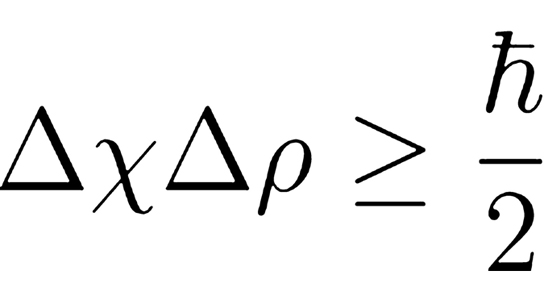## Which equation to use for determining uncertainty?

ALegala_2I
Posts: 102
Joined: Thu Jul 11, 2019 12:17 am

### Which equation to use for determining uncertainty?

Hi,
In the textbook, a modified equation for the uncertainty principle is used with the variable h bar (see below). What does this variable signify? Which equation should we use: the one from the textbook or the one that is taught in class?805097738
Posts: 180
Joined: Wed Sep 18, 2019 12:20 am

### Re: Which equation to use for determining uncertainty?

(uncertainty in position)(uncertainty in momentum)> h/4(pi)

I'd use the formula he taught us in class as Lavelle teaches material and formulas relevant to his tests

Wendy 1E
Posts: 111
Joined: Sat Aug 17, 2019 12:17 am
Been upvoted: 2 times

### Re: Which equation to use for determining uncertainty?

h bar is a simplified form of Planck's constant, in which h bar is equal to h/2π. If you use the equation given in the textbook and substitute h bar as h/2π, you will see the equation that professor Lavelle gave us. This is because (h bar/2π)/2 is equal to h/4π. In regards to your question of which equation to use, I would use the equation that professor Lavelle gave us.

JohnWalkiewicz2J
Posts: 103
Joined: Thu Jul 11, 2019 12:17 am
Been upvoted: 1 time

### Re: Which equation to use for determining uncertainty?

Hey!
So even though Prof Lavelle taught us the formula: (Δp)(Δx) ≥ (h/4π), I found it easier to use the one given in the textbook: (Δp)(Δx) ≥ (1/2)(ћ), when ћ=(h/2π). For some of the textbook problems, using the formula that Prof Lavelle taught in class resulted in the wrong answer, but that was a probably a mistake on my part. Use whatever formula you feel more comfortable with!

Verity Lai 2K
Posts: 100
Joined: Sat Aug 24, 2019 12:18 am

### Re: Which equation to use for determining uncertainty?

The fancy h just means h/2pi and they wrote it that way in the book so it would be shorter and maybe easier to remember but either way works. If you do use the fancy h, you have to remember to put it over an extra 2 so it equals h/4pi.